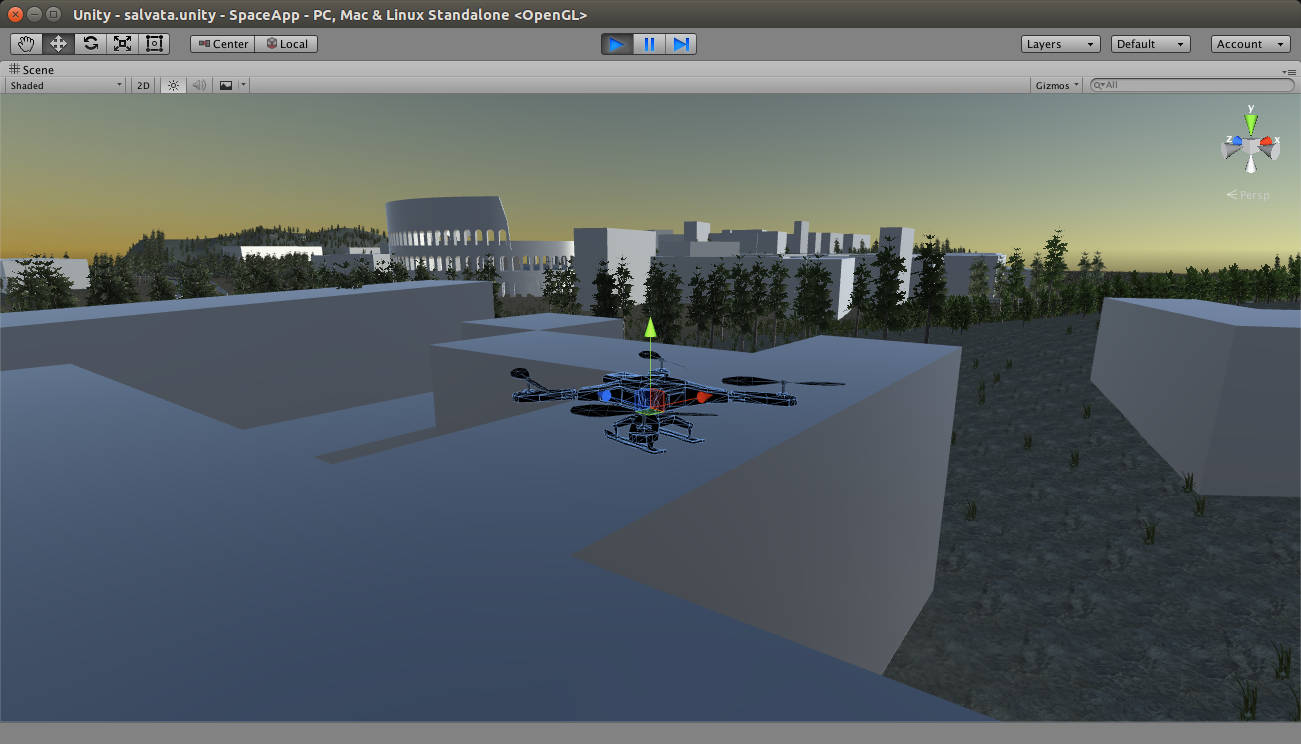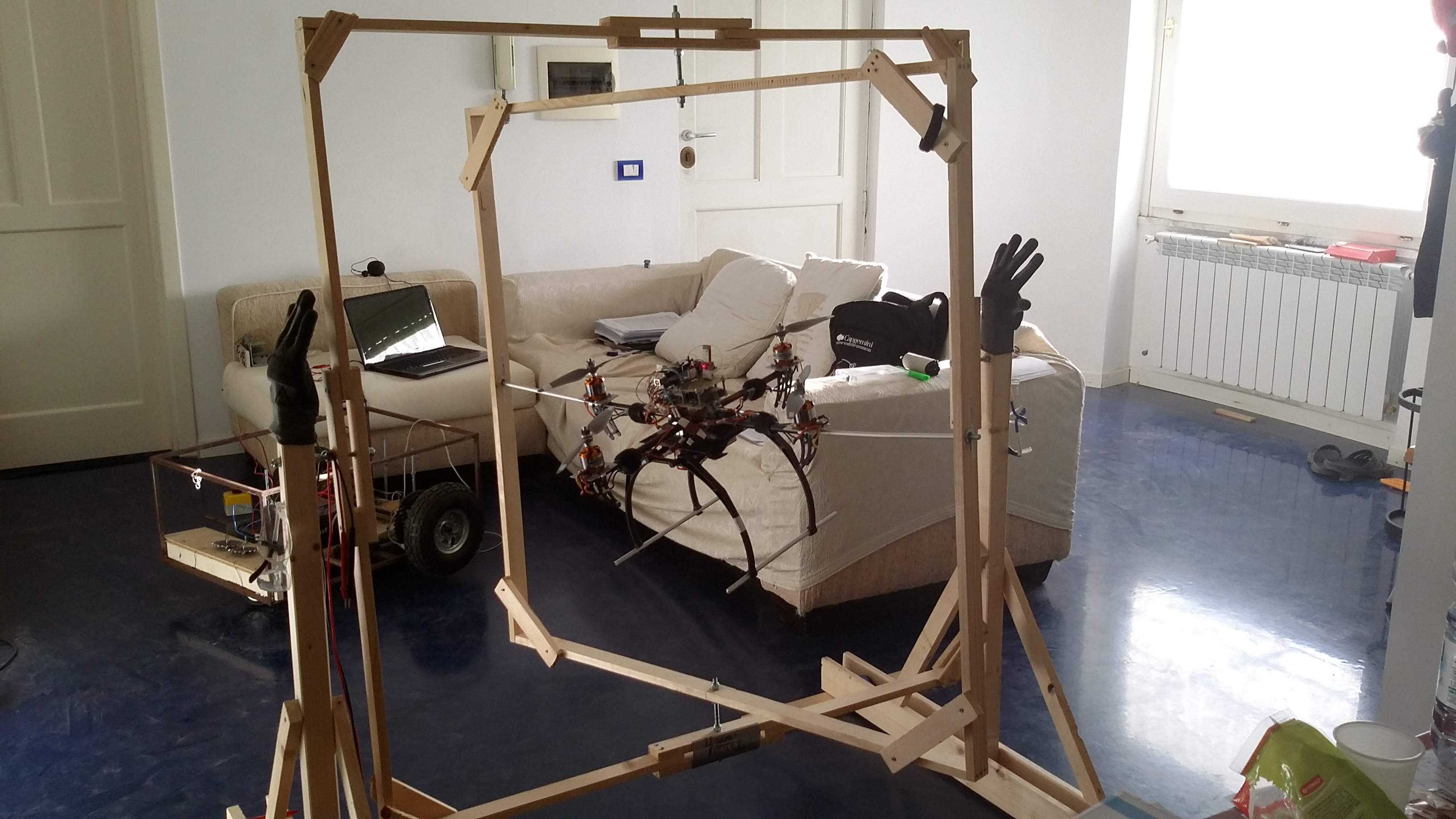•## Python – Scikits Candidate Optimal trajectory

|

On the last article we have installed the Python Scikits library and run the samples provided. In this quick article we would like to find a candidate optimal trajectory that minimizes a weighted combination of speed and position values during a considered time interval \$t_0=0\$ s and \$t_f=10\$s. The performance measure \$J(u)\$ looks like

•## Gyroscope – Filtering angular velocity spikes

|

In this post we will use Matlab to filter angular velocity data acquired with an Arduino. The gyroscope we will be using is a tri-axis L3G4200D sensor. In a previous post - Can you roll with a L3G4200D gyroscope, Arduino and Matlab? we have seen how to configure it and extract the angular rates using